# Troops

The route is long 147 km and the first-day first regiment went at an average speed of 12 km/h and journey back 21 km/h. The second day went second regiment the same route at an average speed of 22 km/h there and back.

Which regiment will take route longer?

Result

#### Solution:

$t_1 = \dfrac{ 147}{ 12} + \dfrac{ 147}{ 21} = 19.25 \ h \ \\ t_2 = \dfrac{ 147}{ 22}+ \dfrac{ 147}{ 22} = 13.36 \ h \ \\ \ \\ t_1 > t_2$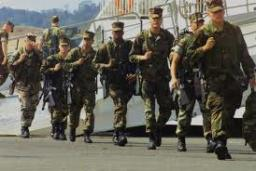We would be very happy if you find an error in the example, spelling mistakes, or inaccuracies, and please send it to us. We thank you!

Showing 1 comment:Math student
3qne=b=3wn8e b=Tips to related online calculators
Need help calculate sum, simplify or multiply fractions? Try our fraction calculator.
Check out our ratio calculator.
Do you have a linear equation or system of equations and looking for its solution? Or do you have quadratic equation?
Do you want to convert length units?
Do you want to convert velocity (speed) units?
Do you want to convert time units like minutes to seconds?

## Next similar math problems:

• Two vases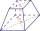Michaela has two vases in her collection. The first vase has the shape of a cone with a base diameter d = 20 cm; the second vase has the shape of a truncated cone with the diameter of the lower base d1 = 25 cm and with the diameter of the upper base d2 =The regular quadrilateral pyramid has a base edge a = 1.56 dm and a height h = 2.05 dm. Calculate: a) the deviation angle of the sidewall plane from the base plane b) deviation angle of the side edge from the plane of the base
• Wooden bowls20 wooden bowls in the shape of a truncated cone should be painted on the outside and inside with wood varnish. We need 0.1 l of paint to paint 200 cm2. How many liters of paint do we have to buy if the bowls are 25 cm high, the bottom of the bowl has a d
• From plasticine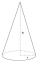Michael modeled from plasticine a 15 cm high pyramid with a rectangular base with the sides of the base a = 12 cm and b = 8 cm. From this pyramid, Janka modeled a rotating cone with a base diameter d = 10 cm. How tall was Janka's cone?
• Cuboid diagonalsThe cuboid has dimensions of 15, 20 and 40 cm. Calculate its volume and surface, the length of the body diagonal and the lengths of all three wall diagonals.
• Find theFind the surface area of a regular quadrilateral pyramid which has a volume of 24 dm3 and a height of 45 cm.
• Rhombus diagonalsIn the rhombus ABCD are given the sizes of diagonals e = 24 cm; f = 10 cm. Calculate the side length of the diamond and the size of the angles, calculate the content of the diamond
• Find the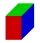Find the surface of the cuboid if its edges have the dimensions a, 2/3a, 2a
• Five circlesOn the line segment CD = 6 there are 5 circles with radius one at regular intervals. Find the lengths of the lines AD, AF, AG, BD, and CE
• Ten persons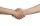Ten persons, each person makes a hand to each person. How many hands were given?
• The bulbThe bulb has a reading of 6V-0.05 A, what current flows through the bulb if we connect it to a cell with a voltage of 12V?
• Isosceles triangleCalculate the area of an isosceles triangle, the base of which measures 16 cm and the arms 10 cm.
• Refractive index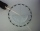The light passes through the interface between air and glass with a refractive index of 1.5. Find: (a) the angle of refraction if light strikes the interface from the air at an angle of 40°. (b) the angle of refraction when light strikes the glass interfaThe height of a regular quadrilateral prism is v = 10 cm, the deviation of the body diagonal from the base is 60°. Determine the length of the base edges, the surface, and the volume of the prism.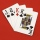What is the probability that we will choose an ace or a king when choosing from a deck of sevens cards?Calculate the surface of the cone if its height is 8 cm and the volume is 301.44 cm3.Calculate the volume of the cone if the content of its base is 78.5 cm2 and the content of the shell is 219.8 cm2.